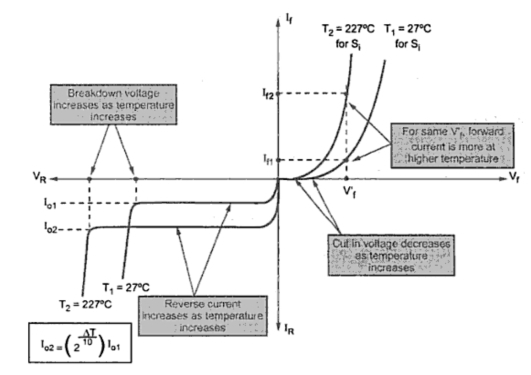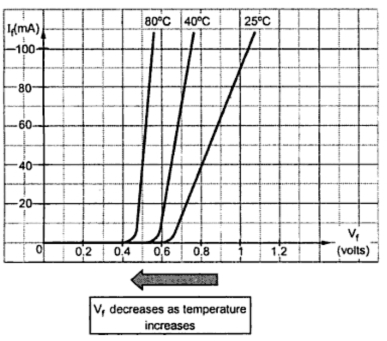### Temperature Dependence p-n Characteristics

The temperature has following effects on the diode parameters,
1. The cut-in voltage decreases as the temperature increases. The diode conducts at smaller voltage at large temperature.
2. The reverse saturation current increases as temperature increases.
This increases in reverse current Io is such that it doubles at every 10 oC rise in temperature. Mathematically,
where              Io2 = Reverse current at T2 oC
Io1 = Reverse current at T1 C
ΔT = (T2 - T1)
3. The voltage equivalent of temperature VT also increases as temperature increases.
4. The reverse breakdown voltage increases as temperature increases.
This is shown in the Fig. 1.Fig. 1  Effect of temperature on diode
1.1 Effect pf Temperature on power dissipation
The diode power dissipation is given by,
To avoid the overheating and damage of the device, the maximum safe value of power dissipation is mentioned in the datasheet of the diode. It is (PD)max. This is specified at normal room temperature of 25 oC.
At higher temperature, as the device junction temperature is higher, it can dissipate less power. Thus maximum power dissipation of the device must be derated at high temperatures.
The Fig. 2 shows power derating graph for a particular device. Upto 25 oC, it is rated for (PD)max of 80 mW but at higher temperatures it is derated as it ca not dissipate same amount of maximum power. For a given temperature, (PD)max value can be directly obtained from the graph. Many a times, a derating factor is provided in the datasheet of the device.
Using this factor, new power P2 at new temperature T2 can be obtained from initial temperature T1 as,Fig. 2 Power derated at high temperature

where          ΔT = T2 - T1 = Temperature rise
Once the new power is known, as Vf is almost constant, new forward current If can be obtained.
1.2 Effect of Temperature on Forward Voltage Drop
Most of the times, the drop across the diode is assumed constant. But in few situations, it is necessary to consider the effect of temperature on forward voltage drop.
It is seen that cut-in voltage decreases as temperature increases.
Note :The diode forward voltage drop decrease as temperature increases.
The rate at which it increases is - 2.3 mV/ oC for silicon while - 2.12 mV/ oC for germanium. The negative sign shows decrease in forward voltage drop as temperature increases.
This is shown in Fig. 3.Fig. 3   Effect of temperature on forward voltage drop

The coefficient ΔVf/ oC is called voltage/temperature coefficient of diode. Knowing this and Vf1 at T1, any Vf2 at T2 can be obtained as,

1.3 Effect of Temperature on Dynamic Resistance
The dynamic resistance of the diode is obtained as,
where k = Boltzman's constant and T in oK constant.
The value 26 mV is temperature dependent and the above equation is applicable only at 25 oC.
For higher temperatures, it gets changed as,
where T' is new temperature in oK.
The above equation can be expressed as,
Note : As temperature increases, VT increase hence dynamic forward resistance increases.